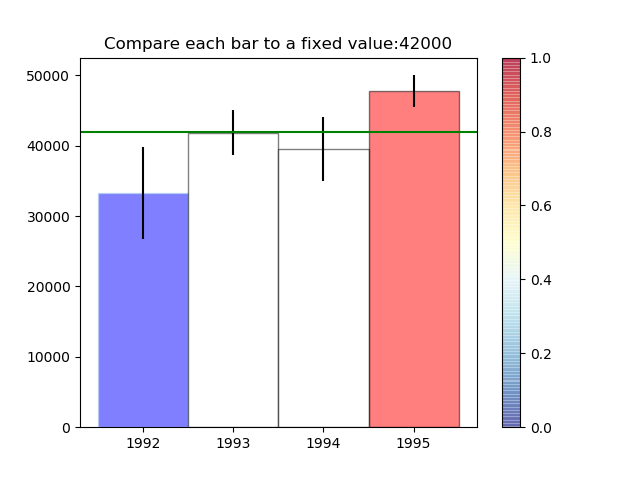# Assignment 3 - Building a Custom Visualization¶

In this assignment you must choose one of the options presented below and submit a visual as well as your source code for peer grading. The details of how you solve the assignment are up to you, although your assignment must use matplotlib so that your peers can evaluate your work. The options differ in challenge level, but there are no grades associated with the challenge level you chose. However, your peers will be asked to ensure you at least met a minimum quality for a given technique in order to pass. Implement the technique fully (or exceed it!) and you should be able to earn full grades for the assignment.

Ferreira, N., Fisher, D., & Konig, A. C. (2014, April). Sample-oriented task-driven visualizations: allowing users to make better, more confident decisions.       In Proceedings of the SIGCHI Conference on Human Factors in Computing Systems (pp. 571-580). ACM. (video)

In this paper the authors describe the challenges users face when trying to make judgements about probabilistic data generated through samples. As an example, they look at a bar chart of four years of data (replicated below in Figure 1). Each year has a y-axis value, which is derived from a sample of a larger dataset. For instance, the first value might be the number votes in a given district or riding for 1992, with the average being around 33,000. On top of this is plotted the 95% confidence interval for the mean (see the boxplot lectures for more information, and the yerr parameter of barcharts).#### Figure 1 from (Ferreira et al, 2014).

A challenge that users face is that, for a given y-axis value (e.g. 42,000), it is difficult to know which x-axis values are most likely to be representative, because the confidence levels overlap and their distributions are different (the lengths of the confidence interval bars are unequal). One of the solutions the authors propose for this problem (Figure 2c) is to allow users to indicate the y-axis value of interest (e.g. 42,000) and then draw a horizontal line and color bars based on this value. So bars might be colored red if they are definitely above this value (given the confidence interval), blue if they are definitely below this value, or white if they contain this value.#### Figure 2c from (Ferreira et al. 2014). Note that the colorbar legend at the bottom as well as the arrows are not required in the assignment descriptions below.

Easiest option: Implement the bar coloring as described above - a color scale with only three colors, (e.g. blue, white, and red). Assume the user provides the y axis value of interest as a parameter or variable.

Harder option: Implement the bar coloring as described in the paper, where the color of the bar is actually based on the amount of data covered (e.g. a gradient ranging from dark blue for the distribution being certainly below this y-axis, to white if the value is certainly contained, to dark red if the value is certainly not contained as the distribution is above the axis).

Even Harder option: Add interactivity to the above, which allows the user to click on the y axis to set the value of interest. The bar colors should change with respect to what value the user has selected.

Hardest option: Allow the user to interactively set a range of y values they are interested in, and recolor based on this (e.g. a y-axis band, see the paper for more details).

Note: The data given for this assignment is not the same as the data used in the article and as a result the visualizations may look a little different.

In :
# Use the following data for this assignment:

import pandas as pd
import numpy as np

np.random.seed(12345)

df = pd.DataFrame([np.random.normal(32000,200000,3650),
np.random.normal(43000,100000,3650),
np.random.normal(43500,140000,3650),
np.random.normal(48000,70000,3650)],
index=[1992,1993,1994,1995])
df

Out:
0 1 2 3 4 5 6 7 8 9 ... 3640 3641 3642 3643 3644 3645 3646 3647 3648 3649
1992 -8941.531897 127788.667612 -71887.743011 -79146.060869 425156.114501 310681.166595 50581.575349 88349.230566 185804.513522 281286.947277 ... 171938.760289 150650.759924 203663.976475 -377877.158072 -197214.093861 24185.008589 -56826.729535 -67319.766489 113377.299342 -4494.878538
1993 -51896.094813 198350.518755 -123518.252821 -129916.759685 216119.147314 49845.883728 149135.648505 62807.672113 23365.577348 -109686.264981 ... -44566.520071 101032.122475 117648.199945 160475.622607 -13759.888342 -37333.493572 103019.841174 179746.127403 13455.493990 34442.898855
1994 152336.932066 192947.128056 389950.263156 -93006.152024 100818.575896 5529.230706 -32989.370488 223942.967178 -66721.580898 47826.269111 ... 165085.806360 74735.174090 107329.726875 199250.734156 -36792.202754 -71861.846997 26375.113219 -29328.078384 65858.761714 -91542.001049
1995 -69708.439062 -13289.977022 -30178.390991 55052.181256 152883.621657 12930.835194 63700.461932 64148.489835 -29316.268556 59645.677367 ... -13901.388118 50173.686673 53965.990717 4128.990173 72202.595138 39937.199964 139472.114293 59386.186379 73362.229590 28705.082908

4 rows × 3650 columns

In :
%matplotlib notebook

df = df.transpose()

Out:
1992 1993 1994 1995
0 -8941.531897 -51896.094813 152336.932066 -69708.439062
1 127788.667612 198350.518755 192947.128056 -13289.977022
2 -71887.743011 -123518.252821 389950.263156 -30178.390991
3 -79146.060869 -129916.759685 -93006.152024 55052.181256
4 425156.114501 216119.147314 100818.575896 152883.621657
In :
dd = df.describe()
dd

Out:
1992 1993 1994 1995
count 3650.000000 3650.000000 3650.000000 3650.000000
mean 33312.107476 41861.859541 39493.304941 47743.550969
std 200630.901553 98398.356203 140369.925240 69781.185469
min -717071.175466 -321586.023683 -450827.613097 -189865.963265
25% -102740.398364 -26628.302213 -57436.397393 1774.555612
50% 29674.931050 43001.976658 41396.781369 49404.322978
75% 167441.838695 108296.577923 137261.713785 94164.333867
max 817505.608159 395586.505068 490091.665037 320826.888044
In :
import math
df_mean = dd.loc['mean']
df_std = dd.loc['std']
length = df.shape
standard_error = df_std / math.sqrt(length)
upper_conf = df_mean + 1.9602 * standard_error
down_conf  = df_mean - 1.9602 * standard_error

In :
interest_val = 42000
bar_color = ['white']*4
for i in range(0,4):
if down_conf.iloc[i] > interest_val:
bar_color[i] = 'red'
elif upper_conf.iloc[i] < interest_val:
bar_color[i] = 'blue'

bar_color

Out:
['blue', 'white', 'white', 'red']
In :
fig, ax = plt.subplots()
ax.bar(np.arange(4), df_mean, 1, color = bar_color, yerr = 1.9602*standard_error, edgecolor='lightblue', alpha = 0.5)
ax.axhline(y = interest_val, color = 'green', label = 'interest value')
ax.set_xticks([0,1,2,3])
ax.set_xticklabels(['1992','1993','1994','1995'])
import matplotlib.cm as cm
import matplotlib.colors as color
cmap = cm.get_cmap('RdYlBu_r')#"seismic_r" "jet"
cmappick = cm.ScalarMappable(cmap=cmap, norm=color.Normalize(vmin=0, vmax=1.0))
cmappick.set_array([])
cbar = plt.colorbar(cmappick, alpha = 0.5)
ax.set_title("Compare each bar to a fixed value:{}".format(interest_val))Out:
Text(0.5,1,'Compare each bar to a fixed value:42000')
In :
cm.get_cmap?

In :
plt.bar?